Test: Basic FET Circuits

# Test: Basic FET Circuits

Test Description

## 15 Questions MCQ Test GATE Electrical Engineering (EE) 2023 Mock Test Series | Test: Basic FET Circuits

Test: Basic FET Circuits for Electronics and Communication Engineering (ECE) 2023 is part of GATE Electrical Engineering (EE) 2023 Mock Test Series preparation. The Test: Basic FET Circuits questions and answers have been prepared according to the Electronics and Communication Engineering (ECE) exam syllabus.The Test: Basic FET Circuits MCQs are made for Electronics and Communication Engineering (ECE) 2023 Exam. Find important definitions, questions, notes, meanings, examples, exercises, MCQs and online tests for Test: Basic FET Circuits below.
Solutions of Test: Basic FET Circuits questions in English are available as part of our GATE Electrical Engineering (EE) 2023 Mock Test Series for Electronics and Communication Engineering (ECE) & Test: Basic FET Circuits solutions in Hindi for GATE Electrical Engineering (EE) 2023 Mock Test Series course. Download more important topics, notes, lectures and mock test series for Electronics and Communication Engineering (ECE) Exam by signing up for free. Attempt Test: Basic FET Circuits | 15 questions in 45 minutes | Mock test for Electronics and Communication Engineering (ECE) preparation | Free important questions MCQ to study GATE Electrical Engineering (EE) 2023 Mock Test Series for Electronics and Communication Engineering (ECE) Exam | Download free PDF with solutions
 1 Crore+ students have signed up on EduRev. Have you?
Test: Basic FET Circuits - Question 1

### In the circuit shown in fig. the transistor parameters are as follows: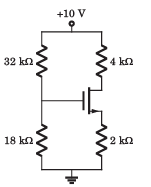Threshold voltage VTN = 2V Conduction parameter Kn = 0.5mA/V2 Que: VGS = ?

Detailed Solution for Test: Basic FET Circuits - Question 1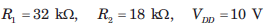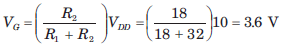Assume that transistor in saturation region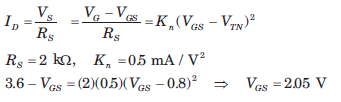Test: Basic FET Circuits - Question 2

### In the circuit shown in fig. the transistor parameters are as follows:Threshold voltage VTN = 2V Conduction parameter Kn = 0.5mA/V2 Que: ID =?

Detailed Solution for Test: Basic FET Circuits - Question 2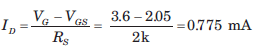Test: Basic FET Circuits - Question 3

### In the circuit shown in fig. the transistor parameters are as follows:Threshold voltage VTN = 2V Conduction parameter Kn = 0.5mA/V2 Que: VDS = ?

Detailed Solution for Test: Basic FET Circuits - Question 3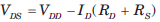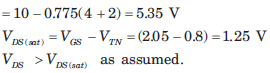Test: Basic FET Circuits - Question 4

In the circuit shown in fig. the transistor parameter are as follows: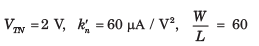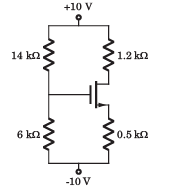Que:

VGS =?

Detailed Solution for Test: Basic FET Circuits - Question 4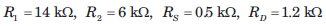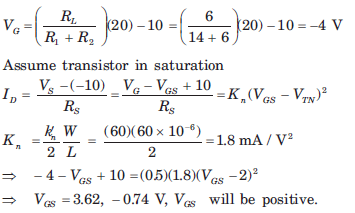Test: Basic FET Circuits - Question 5

In the circuit shown in fig. the transistor parameter are as follows:Que: ID = ?

Detailed Solution for Test: Basic FET Circuits - Question 5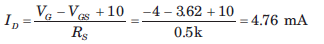Test: Basic FET Circuits - Question 6

The parameter of the transistor in fig.  are VTN = 1.2 V, Kn = 0.5mA / V2 and λ = 0. The voltage VDS is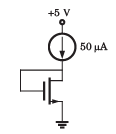Detailed Solution for Test: Basic FET Circuits - Question 6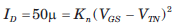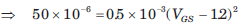VGS = 1.52 V, VGS = VDS

Test: Basic FET Circuits - Question 7

The parameter of the transistor in fig. are VTN = 0.6  V  and Kn = 0.2 mA / V2 . The voltage VS is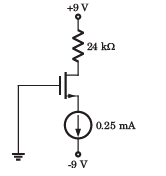Detailed Solution for Test: Basic FET Circuits - Question 7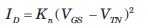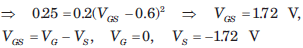Test: Basic FET Circuits - Question 8

In the circuit of fig. the transistor parameters are VTN = 1.7 V and Kn = 0.4mA / V2.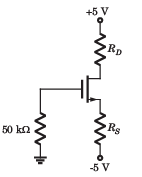If ID = 0.8 mA and VD = 1 V, then value of resistor RS and RD are respectively

Detailed Solution for Test: Basic FET Circuits - Question 8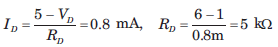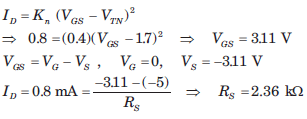Test: Basic FET Circuits - Question 9

In the circuit of fig. the PMOS transistor has parameter VTP = 1.5 V, kp' =  25 μA/V2 , L = 4 μm and λ = 0.If ID = 0.1 mA and VSD = 2.5 V, then value of W will be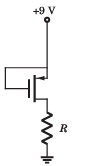Detailed Solution for Test: Basic FET Circuits - Question 9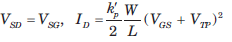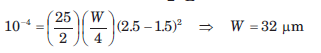Test: Basic FET Circuits - Question 10

The PMOS transistor in fig. has parameters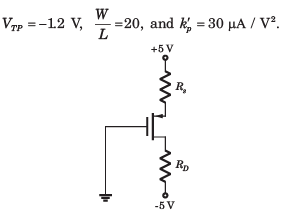Detailed Solution for Test: Basic FET Circuits - Question 10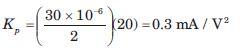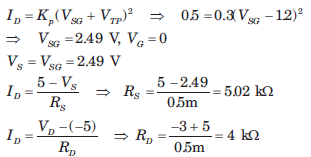Test: Basic FET Circuits - Question 11

The parameters for the transistor in circuit of fig. are VTN = 2 V and Kn = 0.2mA / V2. The power dissipated in the transistor is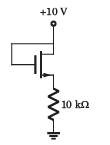Detailed Solution for Test: Basic FET Circuits - Question 11

Assume transistor in saturation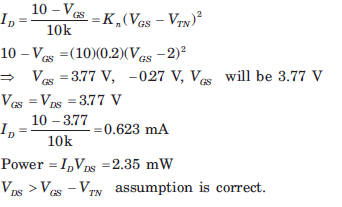Test: Basic FET Circuits - Question 12

Consider the circuit shown in fig.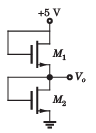The both transistor have parameter as follows

VTN = 0.8V, kn' = 30 μA/V2

Que: If the width-to-length ratios of M1 and M2 are

(W/L)1 = (W/L)2 = 40

The output Vo is

Detailed Solution for Test: Basic FET Circuits - Question 12

For both transistor VDS = VGS ,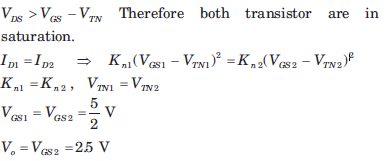Test: Basic FET Circuits - Question 13

Consider the circuit shown in fig.The both transistor have parameter as follows

VTN = 0.8V, kn' = 30 μA/V2

Que: If the ratio is (W/L)1 = 40 and (W/L)2 = 15, then v0 is

Detailed Solution for Test: Basic FET Circuits - Question 13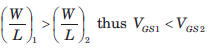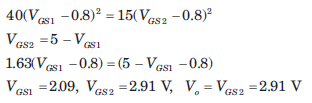Test: Basic FET Circuits - Question 14

In the circuit of fig. the transistor parameters are VTN =1 V and kn' = 36 μA/ V2 .If ID = 0.5 mA, V1 = 5 V and V= 2 V then the width to-length ratio required in each transistor is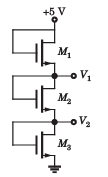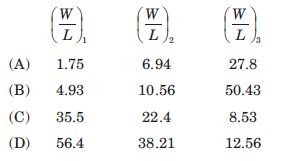Detailed Solution for Test: Basic FET Circuits - Question 14

Each transistor is biased in saturation because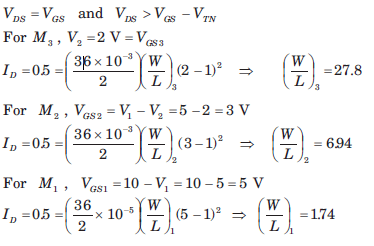Test: Basic FET Circuits - Question 15

The transistors in the circuit of fig. have parameter  VTN = 0.8V, kn' = 40 μA/V2 and λ = 0 .The width-to-length ratio of M2 is (W/L)2 = 1. If Vo = 0.10V when vi = 5 V, then (W/L)1 for M1 is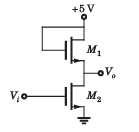Detailed Solution for Test: Basic FET Circuits - Question 15

M2 is in saturation because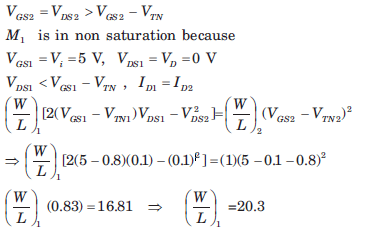## GATE Electrical Engineering (EE) 2023 Mock Test Series

22 docs|274 tests
Information about Test: Basic FET Circuits Page
In this test you can find the Exam questions for Test: Basic FET Circuits solved & explained in the simplest way possible. Besides giving Questions and answers for Test: Basic FET Circuits, EduRev gives you an ample number of Online tests for practice

## GATE Electrical Engineering (EE) 2023 Mock Test Series

22 docs|274 tests(Scan QR code)